# Battista Biggio @ AISec 2013 - Is Data Clustering in Adversarial Settings Secure?

Nov. 4, 2013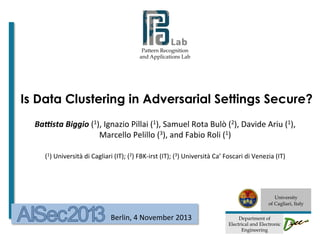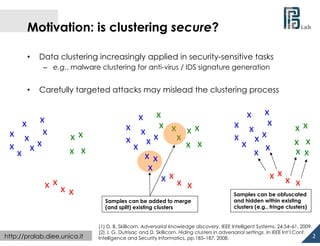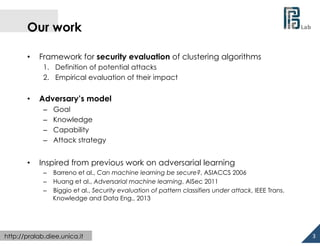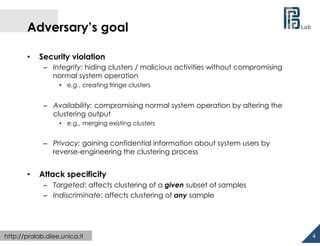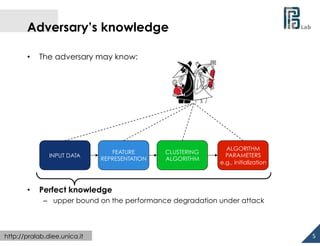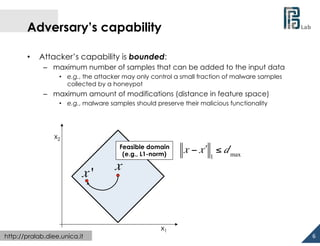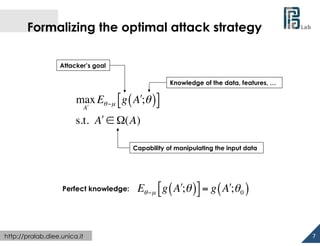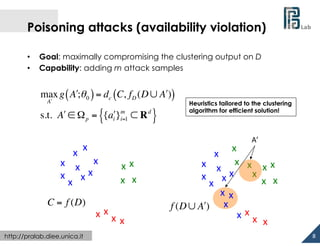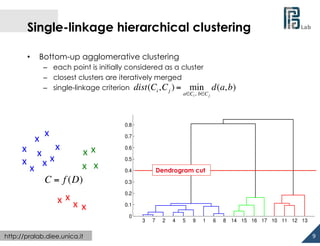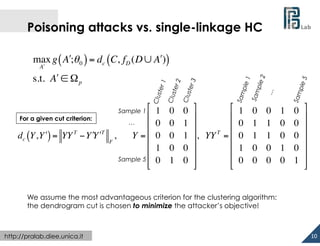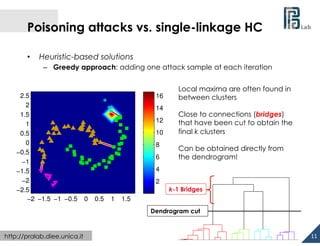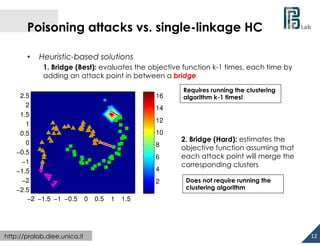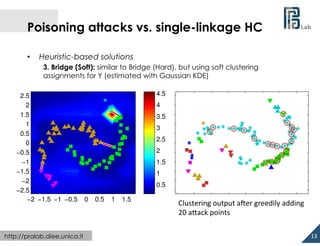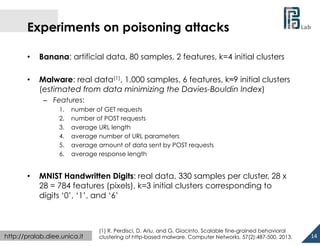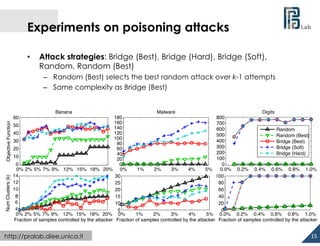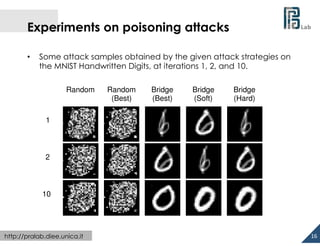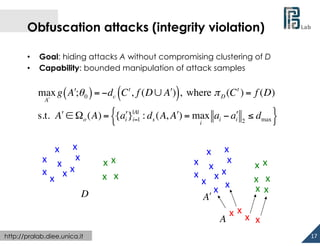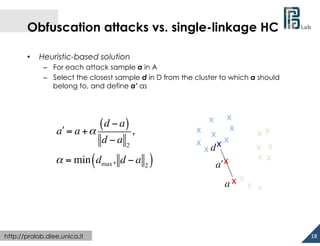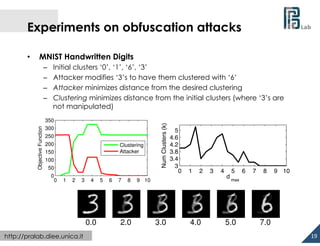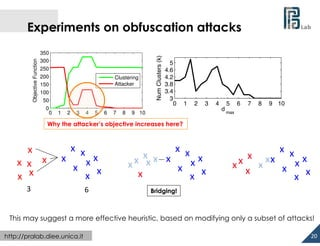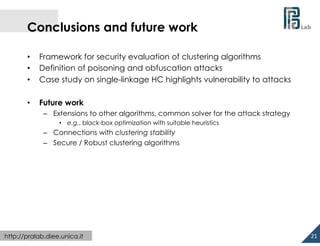1 of 22

### Battista Biggio @ AISec 2013 - Is Data Clustering in Adversarial Settings Secure?

1. Pattern Recognition and Applications Lab Is Data Clustering in Adversarial Settings Secure? Ba#sta  Biggio  (1),  Ignazio  Pillai  (1),  Samuel  Rota  Bulò  (2),  Davide  Ariu  (1),   Marcello  Pelillo  (3),  and  Fabio  Roli  (1)     (1)  Università  di  Cagliari  (IT);  (2)  FBK-­‐irst  (IT);  (3)  Università  Ca’  Foscari  di  Venezia  (IT)                                     Berlin,  4  November  2013   University of Cagliari, Italy   Department of Electrical and Electronic Engineering
2.   Motivation: is clustering secure? •  Data clustering increasingly applied in security-sensitive tasks –  e.g., malware clustering for anti-virus / IDS signature generation •  Carefully targeted attacks may mislead the clustering process x   x   x   x   x   x   x   x   x   x   x   x   x   x   x   x   x   x   x   x   x   x   x   x   x   x   x   x   x   x   x   x   x   x   x   x   x   x   x   Samples can be added to merge (and split) existing clusters http://pralab.diee.unica.it x   x   x   x   x   x   x   x   x   x   x   x   x   x   x   x   x   x   x   x   x   Samples can be obfuscated and hidden within existing clusters (e.g., fringe clusters) (1) D. B. Skillicorn. Adversarial knowledge discovery. IEEE Intelligent Systems, 24:54–61, 2009. (2) J. G. Dutrisac and D. Skillicorn. Hiding clusters in adversarial settings. In IEEE Int’l Conf. 2   Intelligence and Security Informatics, pp.185–187, 2008.
3.   Our work •  Framework for security evaluation of clustering algorithms 1.  Definition of potential attacks 2.  Empirical evaluation of their impact •  Adversary’s model –  –  –  –  •  Goal Knowledge Capability Attack strategy Inspired from previous work on adversarial learning –  Barreno et al., Can machine learning be secure?, ASIACCS 2006 –  Huang et al., Adversarial machine learning, AISec 2011 –  Biggio et al., Security evaluation of pattern classifiers under attack, IEEE Trans. Knowledge and Data Eng., 2013 http://pralab.diee.unica.it 3
4.   Adversary’s goal •  Security violation –  Integrity: hiding clusters / malicious activities without compromising normal system operation •  e.g., creating fringe clusters –  Availability: compromising normal system operation by altering the clustering output •  e.g., merging existing clusters –  Privacy: gaining confidential information about system users by reverse-engineering the clustering process •  Attack specificity –  Targeted: affects clustering of a given subset of samples –  Indiscriminate: affects clustering of any sample http://pralab.diee.unica.it 4
5.   Adversary’s knowledge •  The adversary may know: INPUT DATA •  FEATURE REPRESENTATION CLUSTERING ALGORITHM ALGORITHM PARAMETERS e.g., initialization Perfect knowledge –  upper bound on the performance degradation under attack http://pralab.diee.unica.it 5
6.   Adversary’s capability •  Attacker’s capability is bounded: –  maximum number of samples that can be added to the input data •  e.g., the attacker may only control a small fraction of malware samples collected by a honeypot –  maximum amount of modifications (distance in feature space) •  e.g., malware samples should preserve their malicious functionality x2   Feasible domain (e.g., L1-norm) x' http://pralab.diee.unica.it x x1   x − x " ≤ d max 1 6
7.   Formalizing the optimal attack strategy Attacker’s goal Knowledge of the data, features, … max Eθ ~µ "g ( A!;θ )\$ # % A! s.t. A! ∈ Ω(A) Capability of manipulating the input data Perfect knowledge: http://pralab.diee.unica.it Eθ ~µ "g ( A!;θ )\$ = g ( A!;θ 0 ) # % 7
8.   Poisoning attacks (availability violation) •  •  Goal: maximally compromising the clustering output on D Capability: adding m attack samples max g ( A!;θ 0 ) = dc (C, fD (D ∪ A!)) A! m s.t. A! ∈ Ω p = {{ai!}i=1 ⊂ R d } x   x   x   x   x   x   x   x   x   C = f (D) http://pralab.diee.unica.it x   x   x   Heuristics tailored to the clustering algorithm for efficient solution! A’   x   x   x   x   x   x   x   x   x   x   x   x   x   x   x   x   x   x   x   x   x   x   f (D ∪ A") x   x   x   x   x   8
9.   Single-linkage hierarchical clustering •  Bottom-up agglomerative clustering –  each point is initially considered as a cluster –  closest clusters are iteratively merged –  single-linkage criterion dist(Ci , C j ) = min a∈Ci , b∈C j x   x   x   x   x   x   x   x   x   x   x   x   x   C = f (D) x   x   d(a, b) 0.8 0.7 0.6 0.5 Dendrogram cut 0.4 0.3 0.2 x   x   0.1 0 3 http://pralab.diee.unica.it 7 2 4 5 9 1 6 8 14 15 16 17 10 11 12 13 9
10.   Poisoning attacks vs. single-linkage HC max g ( A!;θ 0 ) = dc (C, fD (D ∪ A!)) A! s.t. A! ∈ Ω p # % For a given cut criterion: … % T T dc (Y, Y !) = YY − Y !Y ! , Y =% F % % Sample 5 % \$ Sample 1 1 0 0 1 0 0 0 0 0 1 0 1 1 0 0 & # 1 0 ( % ( % 0 1 (, YY T = % 0 1 ( % 1 0 ( % ( % 0 0 ' \$ 0 1 1 0 0 1 0 0 1 0 0 0 0 0 1 & ( ( ( ( ( ( ' We assume the most advantageous criterion for the clustering algorithm: the dendrogram cut is chosen to minimize the attacker’s objective! http://pralab.diee.unica.it 10
11.   Poisoning attacks vs. single-linkage HC •  Heuristic-based solutions –  Greedy approach: adding one attack sample at each iteration 2.5 2 1.5 1 0.5 0 −0.5 −1 −1.5 −2 −2.5 −2 −1.5 −1 −0.5 Local maxima are often found in between clusters 16 14 Close to connections (bridges) that have been cut to obtain the final k clusters 12 10 8 Can be obtained directly from the dendrogram! 6 4 0.9 2 0.8 k-1 Bridges 0.7 0 0.5 1 1.5 0.6 0.5 0.4 Dendrogram cut 0.3 0.2 http://pralab.diee.unica.it 0.1 11   0 3 7 9 4 2 5 1 8 6 14 16 17 15 18 21 19 20 10 12 11 13
12.   Poisoning attacks vs. single-linkage HC •  Heuristic-based solutions 1. Bridge (Best): evaluates the objective function k-1 times, each time by adding an attack point in between a bridge 2.5 2 1.5 1 0.5 0 −0.5 −1 −1.5 −2 −2.5 −2 −1.5 −1 −0.5 http://pralab.diee.unica.it 16 Requires running the clustering algorithm k-1 times! 14 12 10 8 6 4 2 0 0.5 1 2. Bridge (Hard): estimates the objective function assuming that each attack point will merge the corresponding clusters Does not require running the clustering algorithm 1.5 12
13.   Poisoning attacks vs. single-linkage HC •  Heuristic-based solutions 3. Bridge (Soft): similar to Bridge (Hard), but using soft clustering assignments for Y (estimated with Gaussian KDE) 2.5 2 1.5 1 0.5 0 −0.5 −1 −1.5 −2 −2.5 −2 −1.5 −1 −0.5 http://pralab.diee.unica.it 0 0.5 1 1.5 4.52.5 4 2 3.51.5 1 3 0.5 2.5 0 2 −0.5 1.5 −1 1 −1.5 −2 0.5 −2.5 −2 −1.5 −1 −0.5 0 0.5 1 1.5 Clustering  output  aVer  greedily  adding   20  aXack  points   13
14.   Experiments on poisoning attacks •  Banana: artificial data, 80 samples, 2 features, k=4 initial clusters •  Malware: real data(1), 1,000 samples, 6 features, k≈9 initial clusters (estimated from data minimizing the Davies-Bouldin Index) –  Features: 1.  2.  3.  4.  5.  6.  •  number of GET requests number of POST requests average URL length average number of URL parameters average amount of data sent by POST requests average response length MNIST Handwritten Digits: real data, 330 samples per cluster, 28 x 28 = 784 features (pixels), k=3 initial clusters corresponding to digits ‘0’, ‘1’, and ‘6’ http://pralab.diee.unica.it (1) R. Perdisci, D. Ariu, and G. Giacinto. Scalable fine-grained behavioral clustering of http-based malware. Computer Networks, 57(2):487-500, 2013. 14
15.   Experiments on poisoning attacks •  Attack strategies: Bridge (Best), Bridge (Hard), Bridge (Soft), Random, Random (Best) –  Random (Best) selects the best random attack over k-1 attempts –  Same complexity as Bridge (Best) Banana Malware Digits 180 800 160 700 50 140 600 Random 120 40 500 Random (Best) 100 400 30 Bridge (Best) 80 300 Bridge (Soft) 60 20 200 Bridge (Hard) 40 10 100 20 0 0 0 0% 2% 5% 7% 9% 12% 15% 18% 20% 0% 1% 2% 3% 4% 5% 0.0% 0.2% 0.4% 0.6% 0.8% 1.0% 6 30 100 4 25 80 14 20 60 12 15 40 10 10 20 8 5 0 0% 2% 5% 7% 9% 12% 15% 18% 20% 0% 1% 2% 3% 4% 5% 0.0% 0.2% 0.4% 0.6% 0.8% 1.0% Fraction of samples controlled by the attacker Fraction of samples controlled by the attacker Fraction of samples controlled by the attacker http://pralab.diee.unica.it Objective Function Objective Function Num Clusters (k) Num Clusters (k) Num Clusters (k) Objective Function 60 15
16.   Experiments on poisoning attacks •  Some attack samples obtained by the given attack strategies on the MNIST Handwritten Digits, at iterations 1, 2, and 10. Random Random (Best) Bridge (Best) Bridge (Soft) Bridge (Hard) 1 2 10 http://pralab.diee.unica.it 16
17.   Obfuscation attacks (integrity violation) •  •  Goal: hiding attacks A without compromising clustering of D Capability: bounded manipulation of attack samples max g ( A!;θ 0 ) = −dc (C t , f (D ∪ A!)), where π D (C t ) = f (D) A! { s.t. A! ∈ Ωo (A) = {ai!}|A| : ds (A, A!) = max ai − ai! 2 ≤ dmax i=1 x   x   x   x   x   x   x   x   x   D x   x   x   x   i x   x   x   x   x   x   x   x   x   x   x   A! A http://pralab.diee.unica.it x   x   x   } x   x   x   x   x   x   x   17
18.   Obfuscation attacks vs. single-linkage HC •  Heuristic-based solution –  For each attack sample a in A –  Select the closest sample d in D from the cluster to which a should belong to, and define a’ as ( d − a) , a! = a + α d−a 2 α = min ( dmax , d − a 2 ) x   x   x   x   x   x   x   x   x  d a! x   x   x   x   x   x   x   x   a x   x   x   http://pralab.diee.unica.it 18
19.   Experiments on obfuscation attacks MNIST Handwritten Digits Objective Function –  –  –  –  Initial clusters ‘0’, ‘1’, ‘6’, ‘3’ Attacker modifies ‘3’s to have them clustered with ‘6’ Attacker minimizes distance from the desired clustering Clustering minimizes distance from the initial clusters (where ‘3’s are not manipulated) 350 300 250 200 150 Clustering Attacker 100 50 0 0 1 2 3 4 0.0 http://pralab.diee.unica.it 5 6 7 8 2.0 Num Clusters (k) •  5 4.6 4.2 3.8 3.4 3 0 1 2 9 10 3.0 4.0 3 4 5 6 d max 5.0 7 8 9 10 7.0 19
20.   350 300 250 200 150 Clustering Attacker 100 50 0 0 1 2 3 4 5 6 7 8 Num Clusters (k) Objective Function Experiments on obfuscation attacks 5 4.6 4.2 3.8 3.4 3 0 1 2 3 9 10 4 5 6 d max 7 8 9 10 Why the attacker’s objective increases here? x   x   x   x   x   x   x   x   x x   x   x   x   x   3   6   x   x   x x x   x   x  x   x   x   x   x   x   x   x   x   x   x   x  x x x   x   x   x   x   x   x   Bridging! This may suggest a more effective heuristic, based on modifying only a subset of attacks! http://pralab.diee.unica.it 20
21.   Conclusions and future work •  •  •  Framework for security evaluation of clustering algorithms Definition of poisoning and obfuscation attacks Case study on single-linkage HC highlights vulnerability to attacks •  Future work –  Extensions to other algorithms, common solver for the attack strategy •  e.g., black-box optimization with suitable heuristics –  Connections with clustering stability –  Secure / Robust clustering algorithms http://pralab.diee.unica.it 21
22.     Thanks  for  your  aXenon!   ?     Any  ques*ons http://pralab.diee.unica.it 22